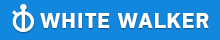GMAT Question of the Day - Daily to your Mailbox; hard ones only

 It is currently 26 Jun 2019, 11:20GMAT Club Daily Prep

Thank you for using the timer - this advanced tool can estimate your performance and suggest more practice questions. We have subscribed you to Daily Prep Questions via email.

Customized
for You

we will pick new questions that match your level based on your Timer History

Track

every week, we’ll send you an estimated GMAT score based on your performance

Practice
Pays

we will pick new questions that match your level based on your Timer History

Not interested in getting valuable practice questions and articles delivered to your email? No problem, unsubscribe here.What is the average (arithmetic mean) of a, b, and c? (1) (a

Author Message
TAGS:

Hide Tags

SVPV
Status: It's near - I can see.
Joined: 13 Apr 2013
Posts: 1688
Location: India
GPA: 3.01
WE: Engineering (Real Estate)
What is the average (arithmetic mean) of a, b, and c? (1) (a  [#permalink]

Show Tags00:00

Difficulty:5% (low)

Question Stats:97% (00:31) correct3% (00:19) wrongbased on 92 sessions

HideShow timer StatisticsWhat is the average (arithmetic mean) of a, b, and c?

(1) (a + b) + (c + d) = 17

(2) d = 5

_________________
"Do not watch clock; Do what it does. KEEP GOING."
ManagerS
Joined: 26 Sep 2018
Posts: 60
Location: Sweden
Re: What is the average (arithmetic mean) of a, b, and c? (1) (a  [#permalink]

Show Tags

1
IMO C.

Average = sum of observations/ number of observations

So a+b+c = 17-d as per Statement 1.
Question arises what is D? So ! alone is insufficient, ruling out A and D.

Statement 2
d=5, on its own it is insufficient. D is not defined by question stem. B is not possible.

So (1)+(2) a+b+c = 17-5 = 12, that works
average = 12/3 =4 as per formula defined above.

C is the correct answer, hence proved.Re: What is the average (arithmetic mean) of a, b, and c? (1) (a   [#permalink] 03 Jan 2019, 15:13
Display posts from previous: Sort by

What is the average (arithmetic mean) of a, b, and c? (1) (a